# Chemistry in Daily Life Questions and Answers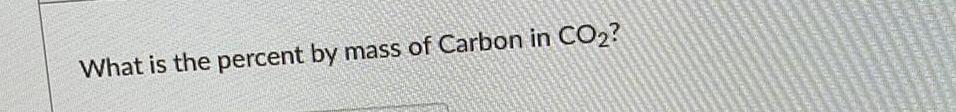Organic Chemistry
Chemistry in Daily Life
What is the percent by mass of Carbon in CO₂?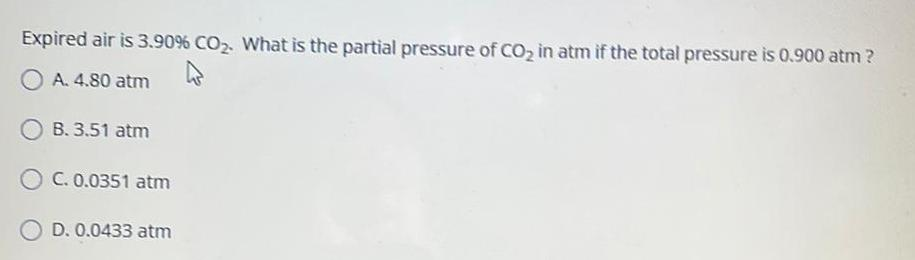Organic Chemistry
Chemistry in Daily Life
Expired air is 3.90% CO₂. What is the partial pressure of CO₂ in atm if the total pressure is 0.900 atm ? A. 4.80 atm B. 3.51 atm C. 0.0351 atm D. 0.0433 atm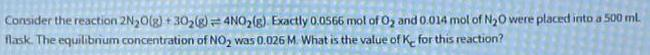Organic Chemistry
Chemistry in Daily Life
Consider the reaction 2N₂O(g) + 30₂(g) = 4NO₂(g) Exactly 0.0566 mol of O₂ and 0.014 mol of N₂0 were placed into a 500 ml. flask. The equilibrium concentration of NO₂ was 0.026 M. What is the value of K for this reaction?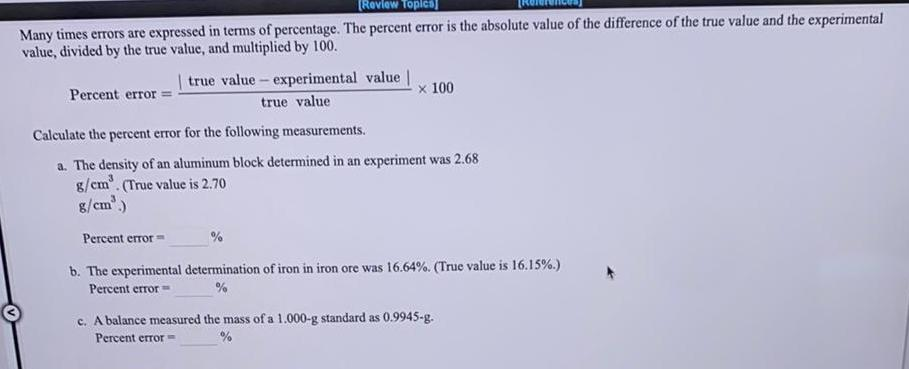Organic Chemistry
Chemistry in Daily Life
Many times errors are expressed in terms of percentage. The percent error is the absolute value of the difference of the true value and the experimental value, divided by the true value, and multiplied by 100. Percent error=|true value-experimental value |/true value x 100 Calculate the percent error for the following measurements. a. The density of an aluminum block determined in an experiment was 2.68 g/cm³. (True value is 2.70 g/cm³.) b. The experimental determination of iron in iron ore was 16.64%. (True value is 16.15%.) Percent error= c. A balance measured the mass of a 1.000-g standard as 0.9945-g. Percent error=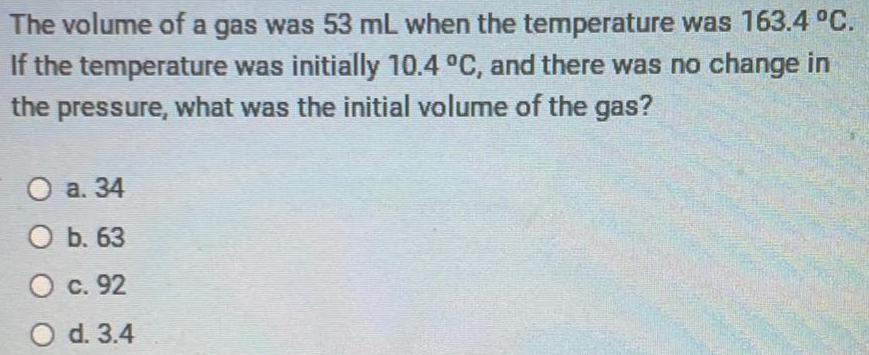Organic Chemistry
Chemistry in Daily Life
The volume of a gas was 53 mL when the temperature was 163.4 °C. If the temperature was initially 10.4 °C, and there was no change in the pressure, what was the initial volume of the gas? a. 34 b. 63 c. 92 d. 3.4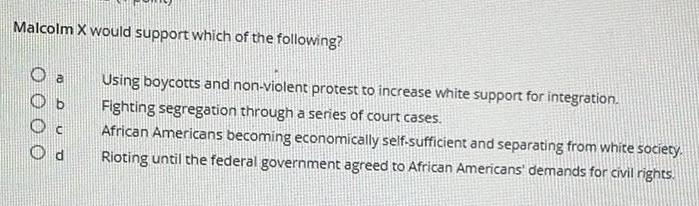Organic Chemistry
Chemistry in Daily Life
Malcolm X would support which of the following? Using boycotts and non-violent protest to increase white support for integration. Fighting segregation through a series of court cases. African Americans becoming economically self-sufficient and separating from white society. Rioting until the federal government agreed to African Americans' demands for civil rights.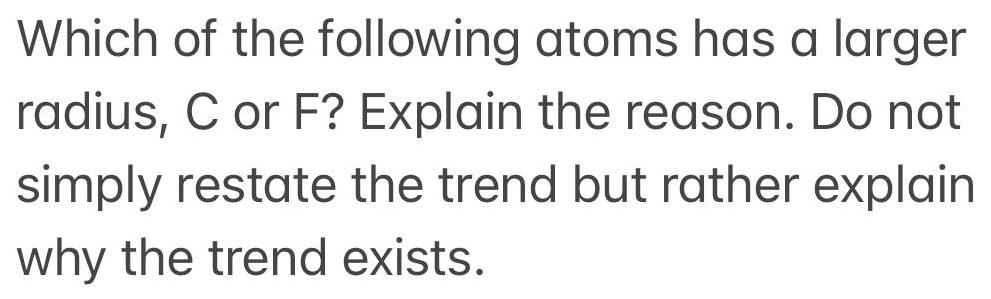Organic Chemistry
Chemistry in Daily Life
Which of the following atoms has a larger radius, C or F? Explain the reason. Do not simply restate the trend but rather explain why the trend exists.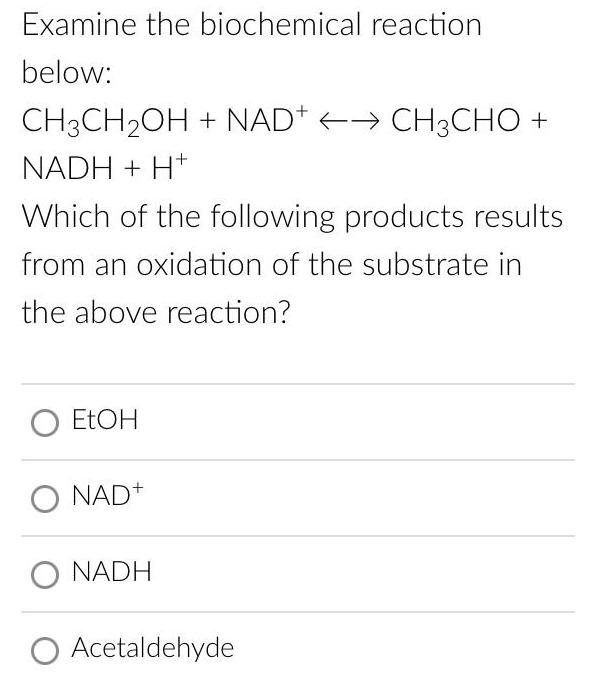Organic Chemistry
Chemistry in Daily Life
Examine the biochemical reaction below: CH3CH₂OH + NAD+ <--> CH3CH+ NADH + H+ Which of the following products results from an oxidation of the substrate in the above reaction? EtOH NAD+ NADH Acetaldehyde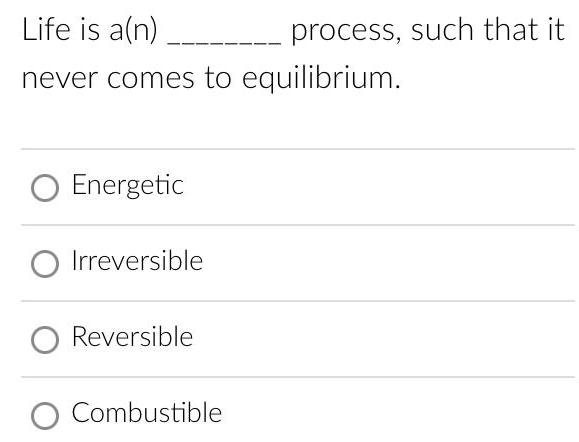Organic Chemistry
Chemistry in Daily Life
Life is a(n) _ never comes to equilibrium. Energetic Irreversible Reversible Combustible process, such that it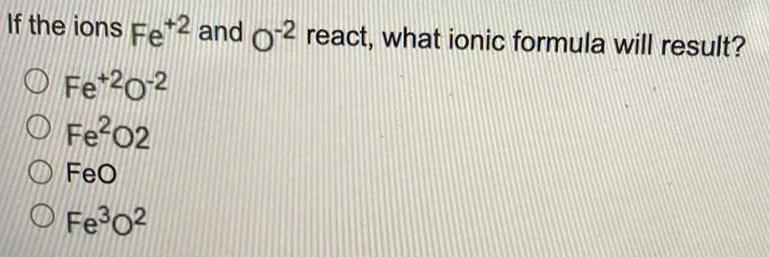Organic Chemistry
Chemistry in Daily Life
If the ions Fe+2 and O-2 react, what ionic formula will result? Fe+2O-2 Fe²O2 FeO Fe³O²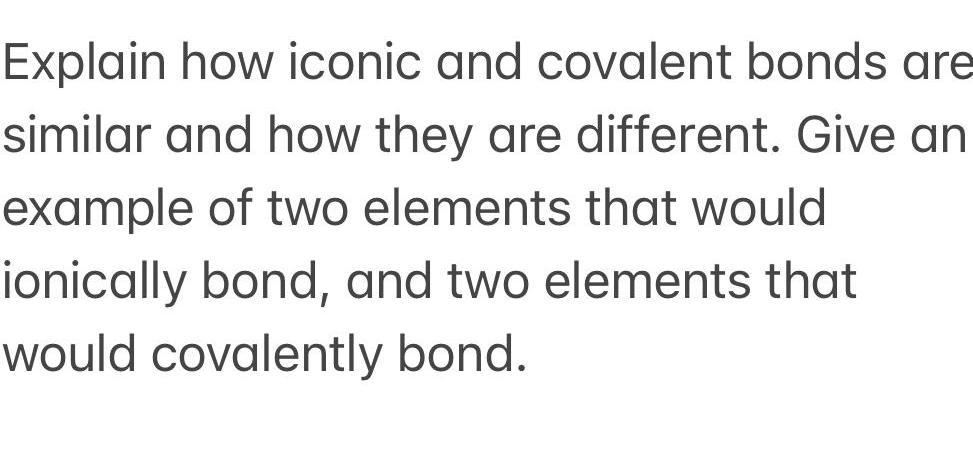Organic Chemistry
Chemistry in Daily Life
Explain how iconic and covalent bonds are similar and how they are different. Give an example of two elements that would ionically bond, and two elements that would covalently bond.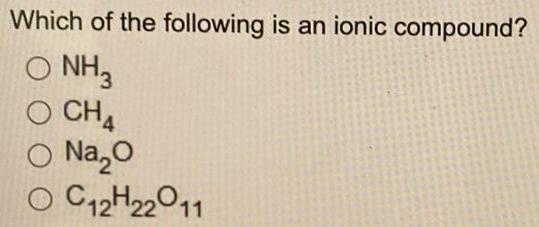Organic Chemistry
Chemistry in Daily Life
Which of the following is an ionic compound? NH3 CH4 Na₂O C12H22011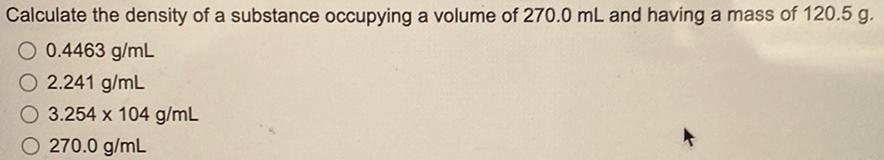Organic Chemistry
Chemistry in Daily Life
Calculate the density of a substance occupying a volume of 270.0 mL and having a mass of 120.5 g. 0.4463 g/mL 2.241 g/mL 3.254 x 104 g/mL 270.0 g/mL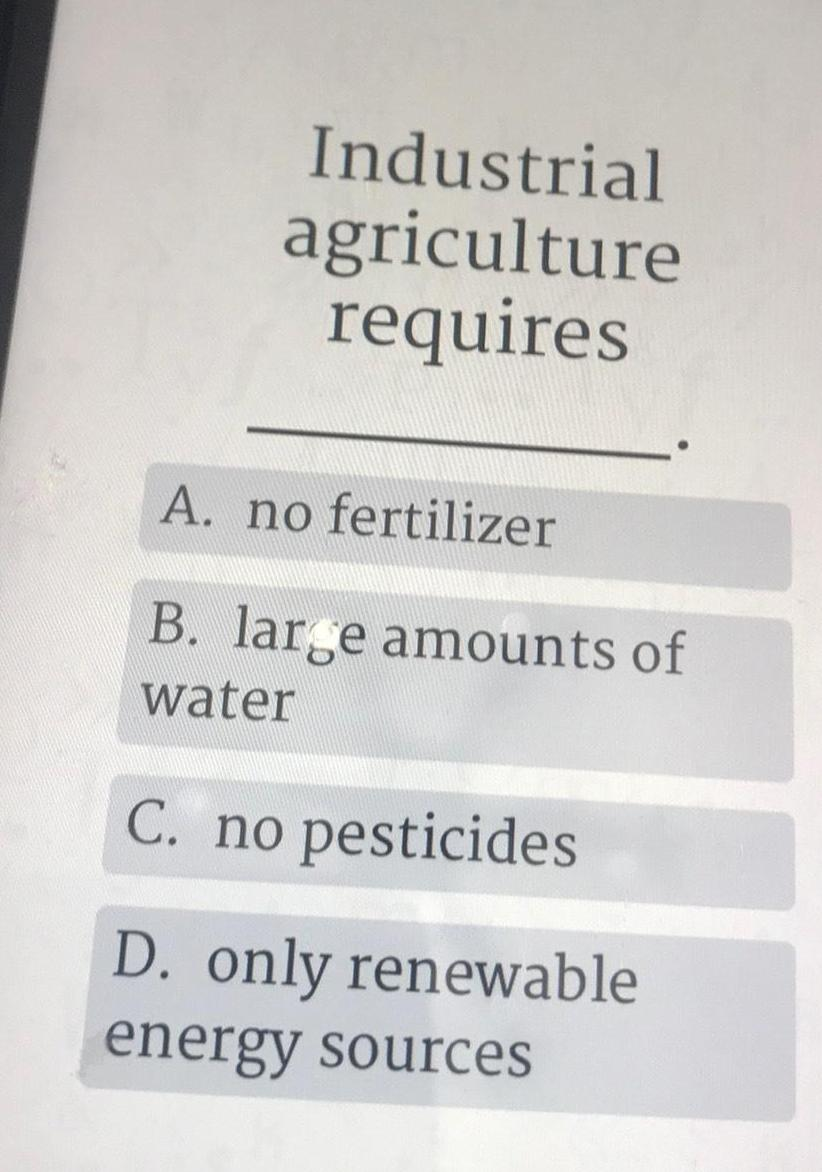Organic Chemistry
Chemistry in Daily Life
Industrial agriculture requires A. no fertilizer B. large amounts of water C. no pesticides D. only renewable energy sources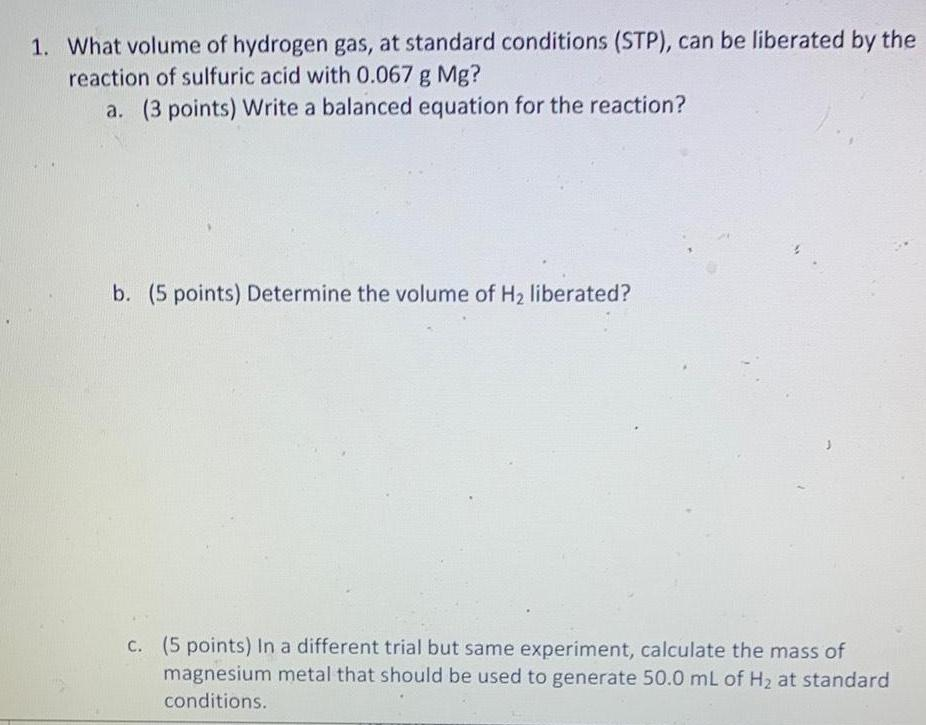Organic Chemistry
Chemistry in Daily Life
What volume of hydrogen gas, at standard conditions (STP), can be liberated by the reaction of sulfuric acid with 0.067 g Mg? Write a balanced equation for the reaction? Determine the volume of H₂ liberated? In a different trial but same experiment, calculate the mass of magnesium metal that should be used to generate 50.0 mL of H₂ at standard conditions.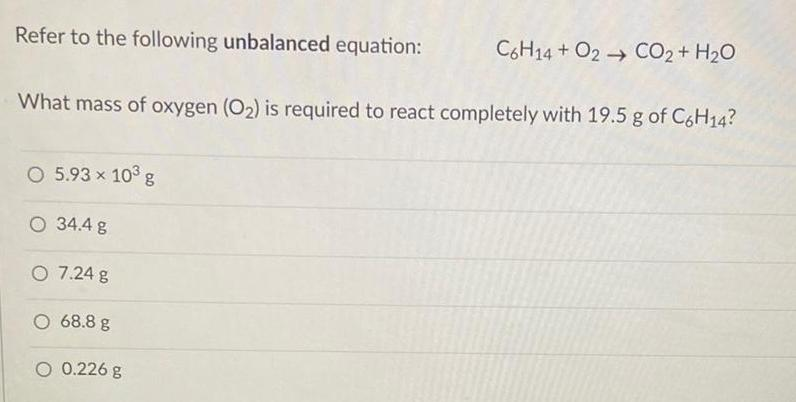Organic Chemistry
Chemistry in Daily Life
Refer to the following unbalanced equation: C6H14+ O2 CO2 + H₂O What mass of oxygen (O₂) is required to react completely with 19.5 g of C6H14? 5.93 × 10³ g 34.4 g 7.24 g 68.8 g 0.226 g